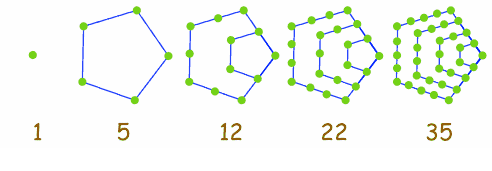# Find the Pentagonal Pyramidal Number using C++

C++Server Side ProgrammingProgramming

#### C in Depth: The Complete C Programming Guide for Beginners

45 Lectures 4.5 hours

#### Practical C++: Learn C++ Basics Step by Step

Most Popular

50 Lectures 4.5 hours

#### Master C and Embedded C Programming- Learn as you go

66 Lectures 5.5 hours

A pentagonal pyramidal number is equal to the number of items in a pentagonal base pyramid. Look at some Pentagonal numbers below.Sum of Pentagonal Numbers till N equals to Nth Pentagonal Pyramidal Number. In this article, we will discuss finding the Nth Pentagonal Pyramidal number, for example

Input : N = 4
Output : 40
Explanation : Sum of first four pentagonal numbers 1, 5, 12, 22 is 40.

Input : N = 6
Output : 126
Explanation : Sum of first four pentagonal numbers 1, 5, 12, 22, 35, 51 is 40.

## Approach to find The Solution

#### Simple Approach

As per the example, the simplest approach comes to mind: traverse the number from 1 to N and keep adding the pentagonal numbers. The pentagonal number can be found by the formula (3 * n2 - n) / 2

e.g For n = 2, pentagonal number = (3 * 22 - 2)/2 = 5

## Example

#include <bits/stdc++.h>
using namespace std;

int main () {
int N = 6, SUM = 0;

// traversing from number 1 to N.
for (int i = 1; i <= N; i++) {
// Calculating ith pentagonal number
// and adding to the SUM.
SUM = SUM + (3 * i * i - i) / 2;
}
cout <<"Nth Pentagonal Pyramidal Number: "<< SUM << endl;
return 0;
}

## Output

Nth Pentagonal Pyramidal Number: 126

## Efficient Approach

The program can be efficient by using a formula to find N Pentagonal Pyramidal Number, which is n2 * (n + 1) / 2.

## Example

#include <bits/stdc++.h>
using namespace std;

int main() {
int N = 6, result;
// calculating Nth pentagonal pyramidal number by formula.
result = N * N * (N + 1) / 2;
cout <<"Nth Pentagonal Pyramidal Number: " << result << endl;
return 0;
}

## Output

Nth Pentagonal Pyramidal Number: 126

## Conclusion

In this article, we discussed the problem of finding the Nth Pentagonal Pyramidal Number. We discussed two approaches to solving this problem: traversing till Nth number and using a formula. We also discussed the C++ program to solve for the same. We can write the same code in other programming languages like C, Java, Python, etc. We hope you find this article helpful.